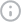0 hidden charges. 0 forex# Price Elasticity of Demand: Definition, Influencing Factors, Formula, & MoreFACT CHECKED
Reviewed by
.
Created on
August 9, 2023## Summary

What’s Inside

The cost of goods and services is always in flux in a market ecosystem due to the rising or declining tendencies of demand and supply. However, to understand what is meant by the price elasticity of demand, we have to focus exclusively on demand itself.

## Understanding the Price Elasticity of Demand

The law of demand states that when the price of a commodity changes, its demand also changes. However, this change varies between different products. For example, products like washing machines and automobiles have a significant change in demand when the price changes. On the other hand, products like fuel and medicines have a minor change in demand. The price elasticity of demand measures how sensitive the demand is to the change in the price of a commodity.

## Factors Influencing Price Elasticity

Multiple factors influence the nature of the commodity, making the demand for some commodities elastic or inelastic.

### 1. Presence of Close Substitutes in the Market

If a product has substitutes, a price increase leads to a decrease in demand as consumers switch to similar products. This makes demand for substitute products more sensitive to price changes, leading to competitive pricing to retain customers. In contrast, products with no substitutes, like electricity, have inelastic demand since consumers have no alternatives.

### 2. Goods of Necessities:

Necessities have inelastic demand, e.g., medications, while luxury goods have more elastic demand, e.g., high-end fashion accessories.

### 3. Income of the Consumers

The income of consumers affects price elasticity of demand. Lower-income consumers are more sensitive to price increases, causing a greater impact on demand and making it more elastic for them. For example, if the price of milk rises, a low-income household would limit their milk consumption, but a high-income household may not be affected as much.

### 4. Time Postponement

Perishable goods have inelastic demand as consumers cannot delay consumption when prices increase. Durable goods have elastic demand because consumers can postpone purchases or buy in bulk when they expect prices to rise. Therefore, many consumers wait for newly launched laptops to drop in price to save money.

### 5. Consumer Habits

Consumer habits can depict the price elasticity of demand too. Loyalty towards a particular brand or product could create an inelasticity of demand, e.g., a large portion of Apple users have high brand loyalty and would opt to buy an Apple product even if its price rises.

## How to Calculate Price Elasticity of Demand?

Formula for calculating the price elasticity of demand is:

 PED = % change in the quantity demanded / % change in price

It can be expanded to the following equation:

 PED = [(Q2 – Q1) / (Q1 + Q2)] ÷ [(P1 – P2) / (P1 + P2)]
• Q1 is the initial quantity demanded.
• Q2 is the quantity demanded after the price change.
• P1 is the initial price.
• P2 is the changed price.

Example of calculating the price elasticity of demand with the formula:

• Let’s assume the initial price (P1) is 24, at which the initial quantity demanded (Q1) is 40, and the changed price (P2) is 12, at which the quantity demanded (Q2) is 80.
• In this case, (Q2 – Q1) is 80 - 40 = 40, and (Q1 + Q2) is 120.
• So, (Q2 – Q1) / (Q1 + Q2) is = 40 / 120 = 1/3 = 33.33%.
• Similarly, (P1 – P2) is 24 - 12 = 12 and (P1 + P2) is 36.
• So, (P1 – P2) / (P1 + P2) is = 12 / 36 = 1/3 = 33.33%
• PED =  33.33% / 33.33%
• PED = 1

The demand is unitarily elastic when the elasticity coefficient (PED) is 1. This means that a 1% change in quantity occurs for every 1% change in price. In the real world, the PED is rarely equal to 1. When the demand is elastic, the elasticity coefficient (PED) is greater than 1, and when the demand is inelastic, the coefficient (PED) is between 0 and 1.

## What is the Cross-Price Elasticity of Demand?

Cross-price elasticity of demand occurs when the demand for one commodity is affected by the price of another. If the demand for masoor dal grows when the price of tur dal goes up, that's positive cross-price elasticity. Conversely, if the demand for ink cartridges goes down when the price of printers goes up, that's negative cross-price elasticity.

## In Conclusion

Price elasticity is important for understanding consumer behavior and market dynamics. It can help with analyzing competition and forecasting revenue. This knowledge can assist businesses, policymakers, economists, and investors in making informed decisions that can impact pricing strategies, profits, and market interventions.

### 1. How is the concept of price elasticity of demand helpful for businesses and policymakers?

It helps businesses to know the impact of price on profitability. It is also useful for policy-making decisions like taxation and regulation.

### 2. Could you provide a real-world example to illustrate different scenarios of price elasticity of demand for various products or services?

When BEST buses in Mumbai reduced the price of their tickets, more travellers started using BEST buses. The demand for salt is an example of the inelasticity of demand. Though the price of salt has increased, demand hasn’t fallen due to it.

### 3. What are the key factors that influence the price elasticity of demand?

The price elasticity of demand mainly depends on the nature of goods and services, the availability of substitute products, the income of the consumers, etc.

# DisclaimerFi Money is not a bank; it offers banking services through licensed partners and investment services through epiFi Wealth Pvt. Ltd. and its partners. This post is for information only and is not professional financial advice.